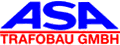# What is an idle currentThe L. denotes the current that flows into the primary side of a transformer when the rated voltage is applied, while the secondary side is unloaded.
He has the formula symbol.

The L. considered here relates to the steady state. In contrast to this, the inrush current describes the transition from the unmagnetized transformer to the no-load current.

The no-load current has an active and a reactive component. The active component, the current of the no-load power loss, is caused by the iron losses, which are divided into hysteresis losses and eddy current losses.

The reactive component arises from the finite main inductance of a real transformer (the magnetic resistance of the core is not 0). The smaller the inductance that is connected to the network, the greater the reactive current that flows. In order to keep the losses in the supply lines low, attempts are made to minimize the no-load current.

In addition, an excessively high no-load current can disturb the network because real networks can be influenced by consumers (no infinitely small internal resistance of the voltage source, line resistance not 0).
The hysteresis curve is not linear and, when linked with the sine wave of the integrated input voltage, leads to a high harmonic current consumption, i.e. the no-load current is not only limited to the mains frequency, but contains spectral components with integer multiples of this frequency.
These additional frequency components can interfere with electronic loads, which are often only protected from interference by filtering out the 50 Hz frequency. However, due to the dimensioning of the networks for a current consumption that is well above the L., the harmonics can usually be neglected.

The effects of the frequency spectrum of the L. on a three-phase transformer depend on its vector group.
By considering the sine functions of the input currents, one comes to the conclusion that the currents of the harmonics with a multiple of the network frequency divisible by three behave like an in-phase load because the three phases are always in the same phase position by multiplying the angle argument by three (120 ° x 3 = 360 °).
In the case of vector group Y on the primary side, this type of behavior leads to an undesirable stray field; with vector group D, the in-phase components of the current can flow freely. Multiples of the mains frequency that cannot be divided by three are either out of phase or just run faster in the same direction of rotation as the input voltage. Both of these cases are unproblematic.

In reality, the L. can fluctuate by up to 30% with identical transformers, because even a very small air gap in the core increases the magnetic resistance significantly. Small manufacturing tolerances therefore lead to a large difference in the no-load current. As long as the L. remains within the permissible range, there are no negative consequences, and the gear ratio hardly changes.